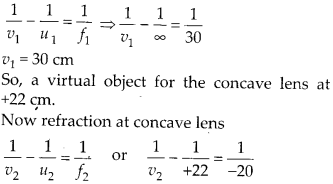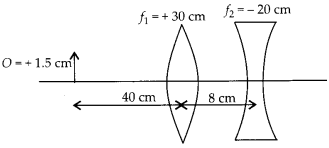Enlightened

# Question 21: NCERT Solutions for 12th Class Physics: Chapter 9-Ray Optics and Optical Instruments

• 0

Question 21: NCERT Solutions for 12th Class Physics: Chapter 9-Ray Optics and Optical Instruments

(a) Determine the ‘effective focal length of the combination of two lenses in question 10, if they are placed 8.0 cm apart with their principal axes coincident. Does the answer depend on which side of the combination a beam of parallel light is incident? Is the notion of effective focal length of this system useful at all?
(b) An object 1.5 cm in size is placed on the side of the convex lens in the above arrangement. The distance between the object and the convex lens is 40 cm. Determine the magnification produced by the two-lens system, and the size of the image.

Share

1. Solution:
(a)
(i) Let a parallel beam of light incident first on convex lens, refraction at convex lensThe parallel beam of light appears to diverge from a point 216 cm from the center of the two lens system.
(ii) Now let a parallel beam of light incident first on concave lens.

The image I1 will act as real object for convex lens at 28 cm.Thus the parallel incident beam appears to diverge from a point 420 -4 = 416 cm on the left of the center of the two lens system. Hence the answer depend upon which side of the lens system the parallel beam is made incident. Therefore the effective focal length is different in two situations.

(b) Now an object of 1.5 cm size is kept 40 cm in front of convex lens in the same system of lenses.Check the complete chapter with solutions.

NCERT Solutions for 12th Class Physics: Chapter 9-Ray Optics and Optical Instruments

• 0